Research papers and code for "Suvrit Sra":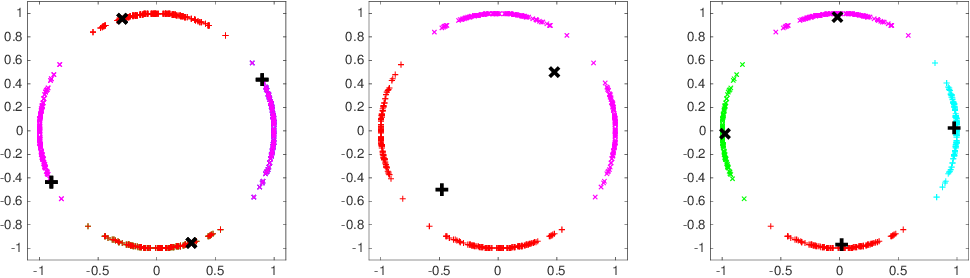The modern data analyst must cope with data encoded in various forms, vectors, matrices, strings, graphs, or more. Consequently, statistical and machine learning models tailored to different data encodings are important. We focus on data encoded as normalized vectors, so that their "direction" is more important than their magnitude. Specifically, we consider high-dimensional vectors that lie either on the surface of the unit hypersphere or on the real projective plane. For such data, we briefly review common mathematical models prevalent in machine learning, while also outlining some technical aspects, software, applications, and open mathematical challenges.

* 12 pages, slightly modified version of submitted book chapter
Click to Read Paper and Get Code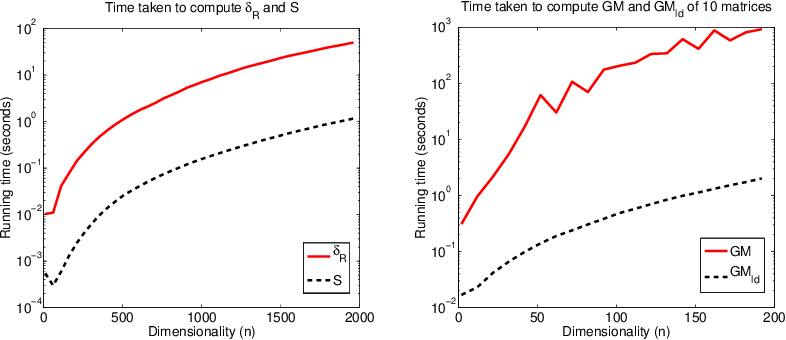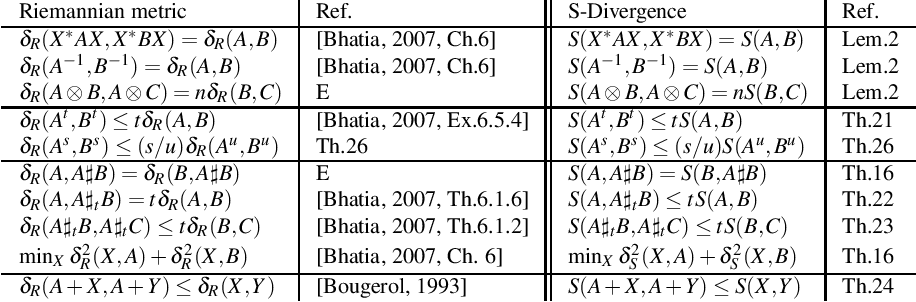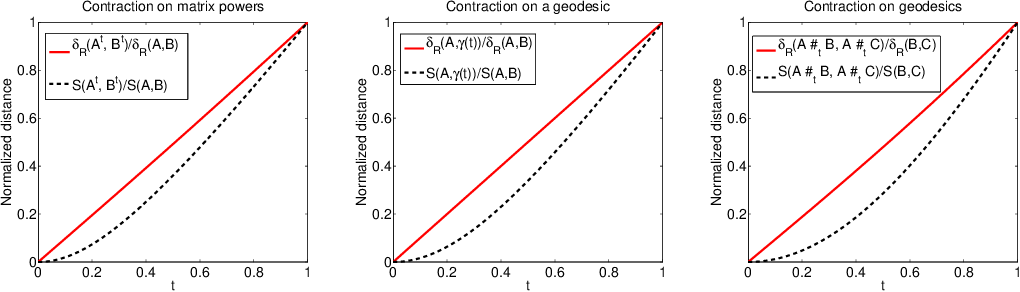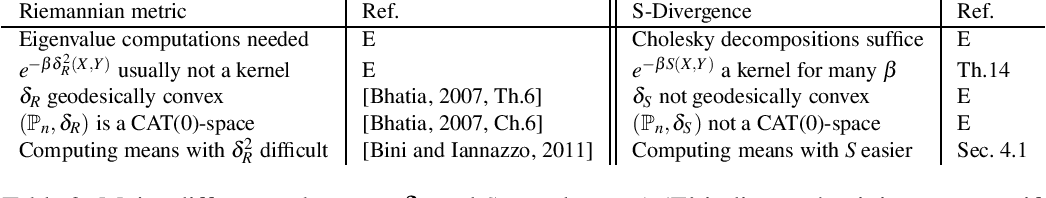Positive definite matrices abound in a dazzling variety of applications. This ubiquity can be in part attributed to their rich geometric structure: positive definite matrices form a self-dual convex cone whose strict interior is a Riemannian manifold. The manifold view is endowed with a "natural" distance function while the conic view is not. Nevertheless, drawing motivation from the conic view, we introduce the S-Divergence as a "natural" distance-like function on the open cone of positive definite matrices. We motivate the S-divergence via a sequence of results that connect it to the Riemannian distance. In particular, we show that (a) this divergence is the square of a distance; and (b) that it has several geometric properties similar to those of the Riemannian distance, though without being computationally as demanding. The S-divergence is even more intriguing: although nonconvex, we can still compute matrix means and medians using it to global optimality. We complement our results with some numerical experiments illustrating our theorems and our optimization algorithm for computing matrix medians.

* 24 pages with several new results; a fraction of this paper also appeared at the Neural Information Processing Systems (NIPS) Conference, Dec. 2012
Click to Read Paper and Get Code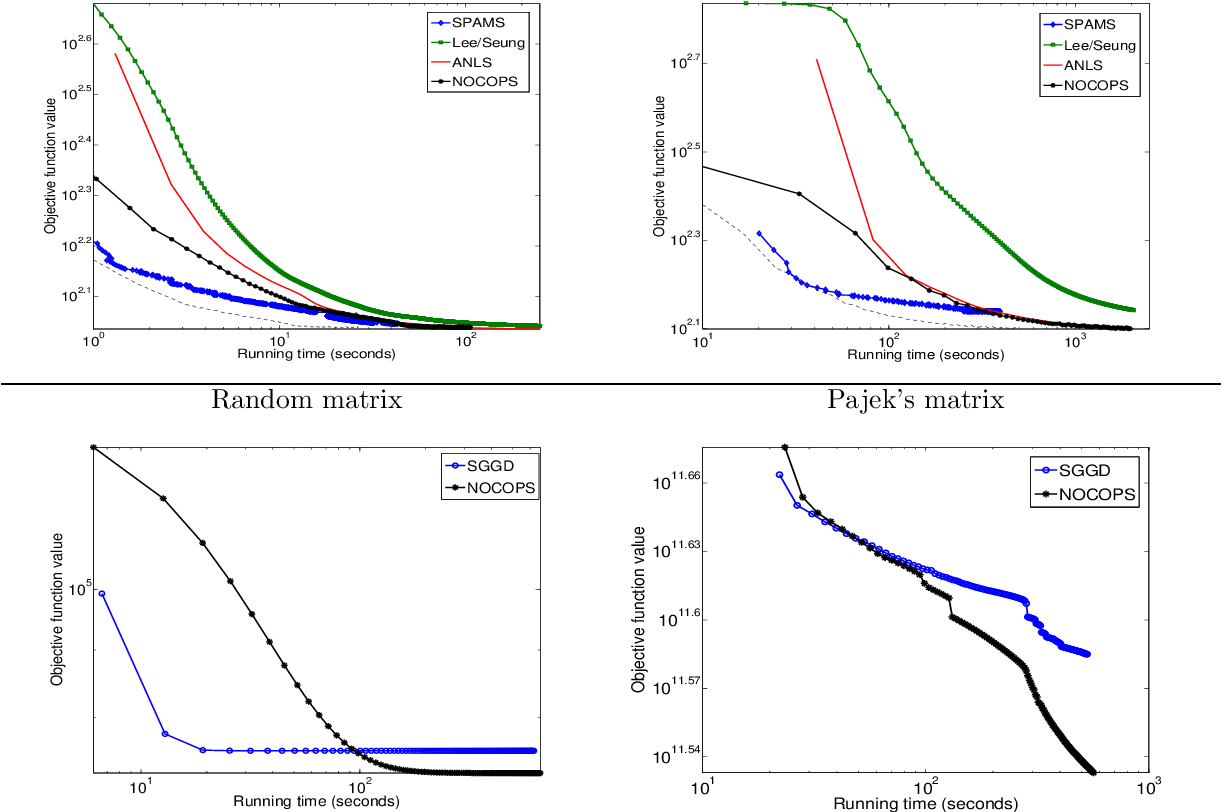Within the unmanageably large class of nonconvex optimization, we consider the rich subclass of nonsmooth problems that have composite objectives---this already includes the extensively studied convex, composite objective problems as a special case. For this subclass, we introduce a powerful, new framework that permits asymptotically non-vanishing perturbations. In particular, we develop perturbation-based batch and incremental (online like) nonconvex proximal splitting algorithms. To our knowledge, this is the first time that such perturbation-based nonconvex splitting algorithms are being proposed and analyzed. While the main contribution of the paper is the theoretical framework, we complement our results by presenting some empirical results on matrix factorization.

* revised version 12 pages, 2 figures; superset of shorter counterpart in NIPS 2012
Click to Read Paper and Get Code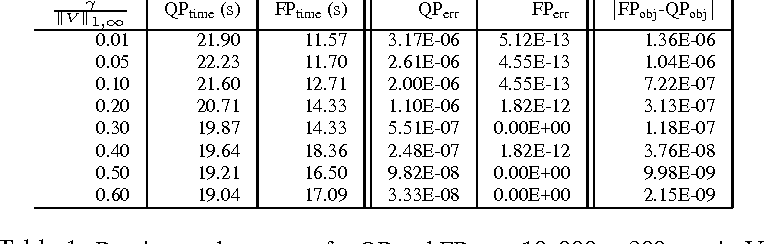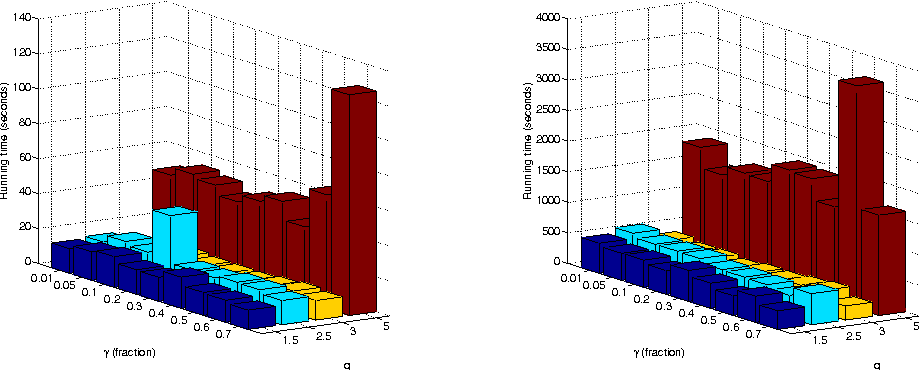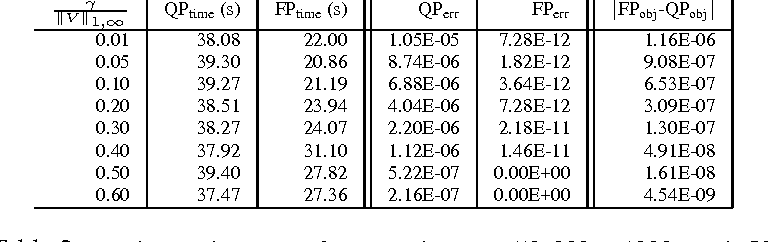Joint sparsity offers powerful structural cues for feature selection, especially for variables that are expected to demonstrate a "grouped" behavior. Such behavior is commonly modeled via group-lasso, multitask lasso, and related methods where feature selection is effected via mixed-norms. Several mixed-norm based sparse models have received substantial attention, and for some cases efficient algorithms are also available. Surprisingly, several constrained sparse models seem to be lacking scalable algorithms. We address this deficiency by presenting batch and online (stochastic-gradient) optimization methods, both of which rely on efficient projections onto mixed-norm balls. We illustrate our methods by applying them to the multitask lasso. We conclude by mentioning some open problems.

* Preprint of paper under review
Click to Read Paper and Get Code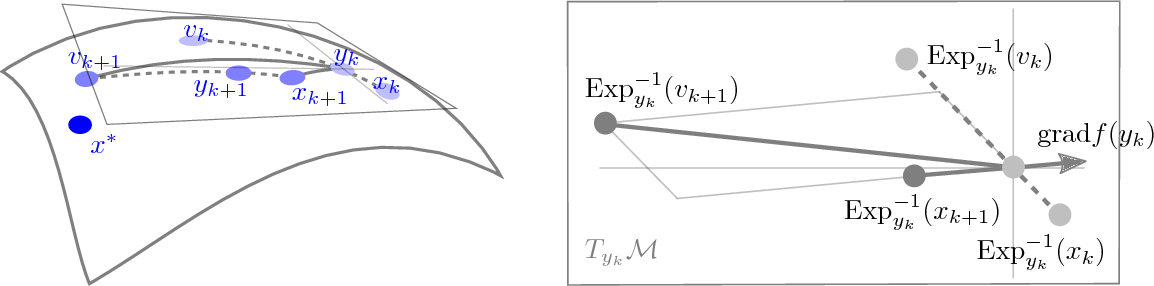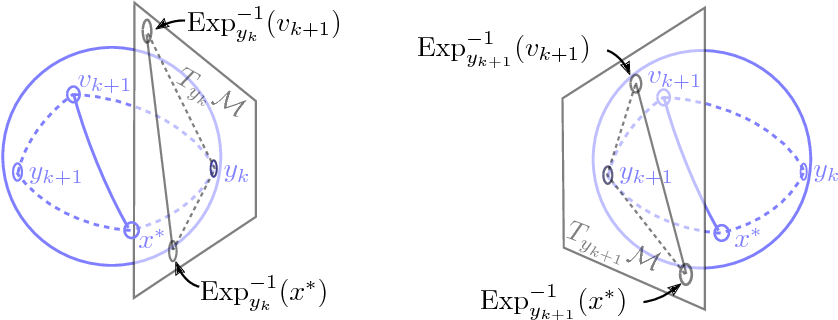We propose a Riemannian version of Nesterov's Accelerated Gradient algorithm (RAGD), and show that for geodesically smooth and strongly convex problems, within a neighborhood of the minimizer whose radius depends on the condition number as well as the sectional curvature of the manifold, RAGD converges to the minimizer with acceleration. Unlike the algorithm in (Liu et al., 2017) that requires the exact solution to a nonlinear equation which in turn may be intractable, our algorithm is constructive and computationally tractable. Our proof exploits a new estimate sequence and a novel bound on the nonlinear metric distortion, both ideas may be of independent interest.

* Published in 31th Annual Conference on Learning Theory (COLT'18)
Click to Read Paper and Get Code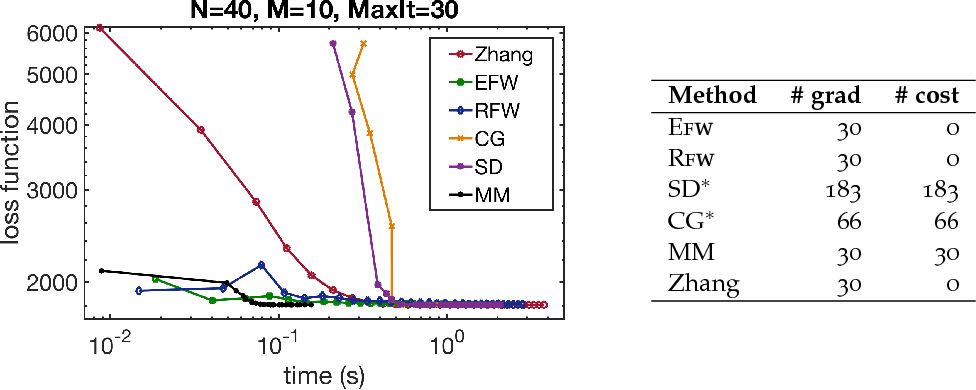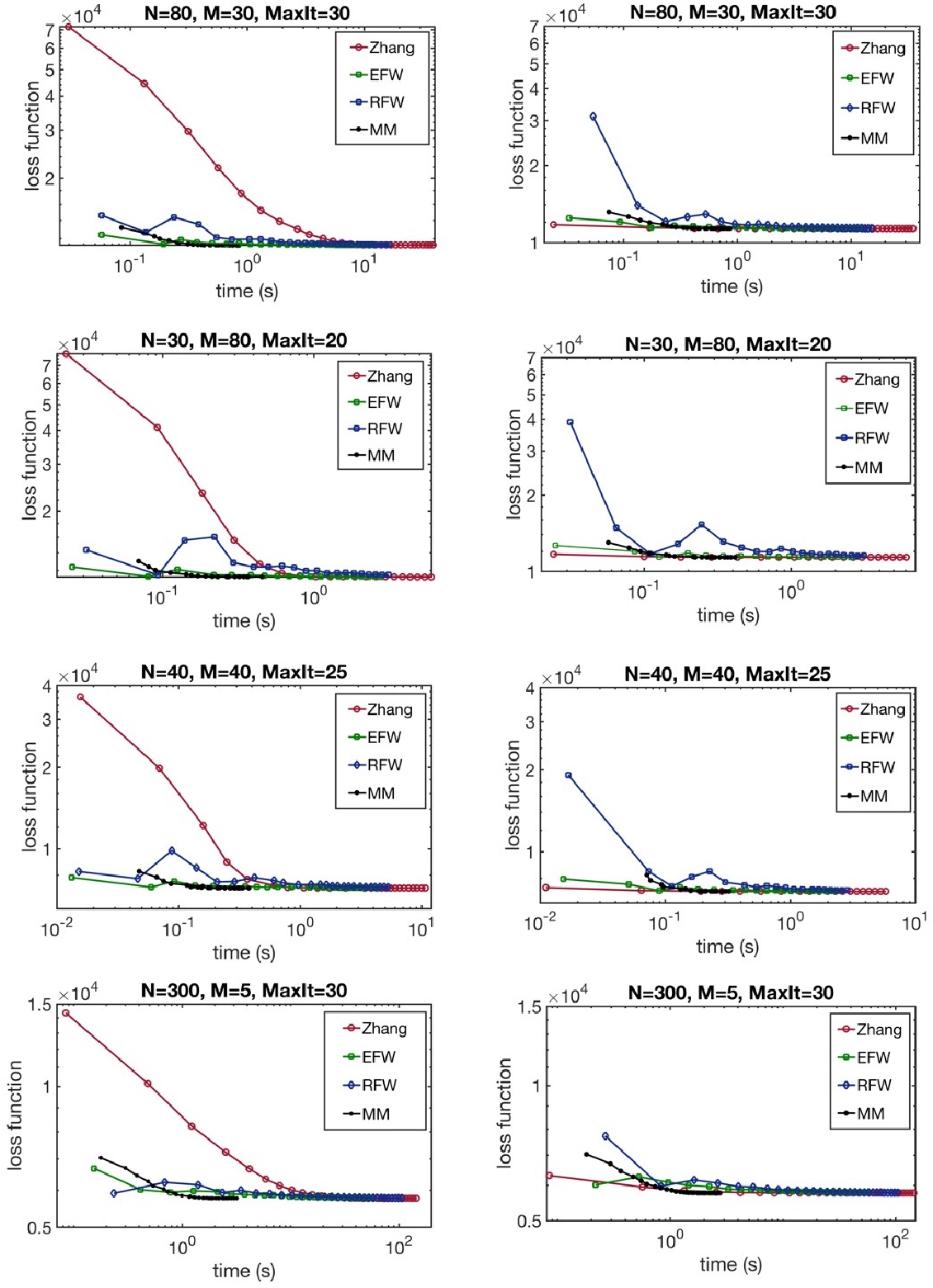We study projection free methods for constrained geodesically convex optimization. In particular, we propose a Riemannian version of the Frank-Wolfe (RFW) method. We analyze RFW's convergence and provide a global, non-asymptotic sublinear convergence rate. We also present a setting under which RFW can attain a linear rate. Later, we specialize RFW to the manifold of positive definite matrices, where we are motivated by the specific task of computing the geometric mean (also known as Karcher mean or Riemannian centroid). For this task, RFW requires access to a "linear oracle" that turns out to be a nonconvex semidefinite program. Remarkably, this nonconvex program is shown to admit a closed form solution, which may be of independent interest too. We complement this result by also studying a nonconvex Euclidean Frank-Wolfe approach, along with its global convergence analysis. Finally, we empirically compare Rfw against recently published methods for the Riemannian centroid and observe strong performance gains.

* Under review; 21 pages, 2 figures
Click to Read Paper and Get Code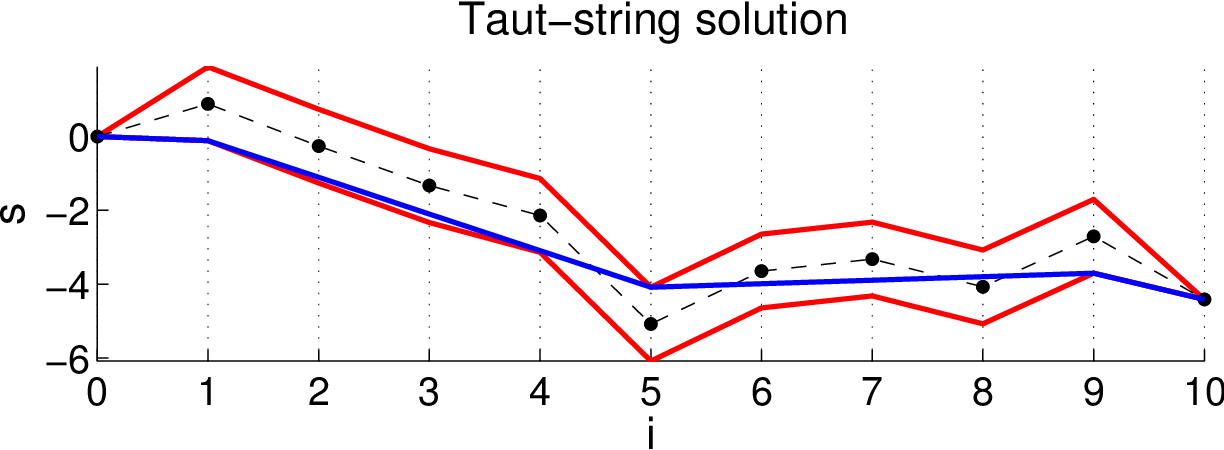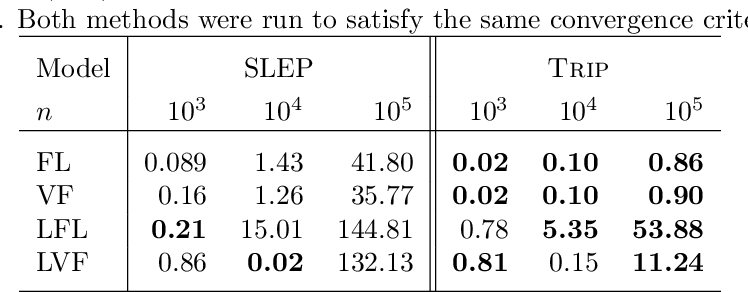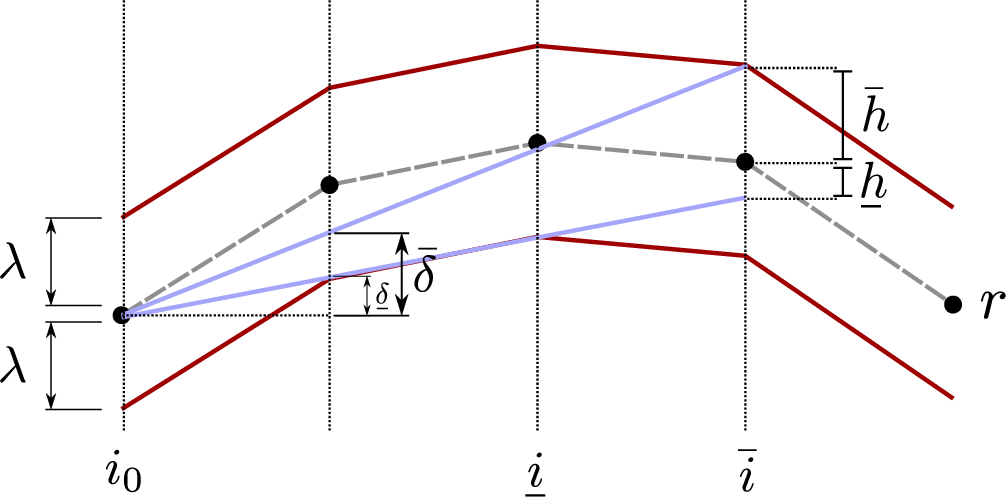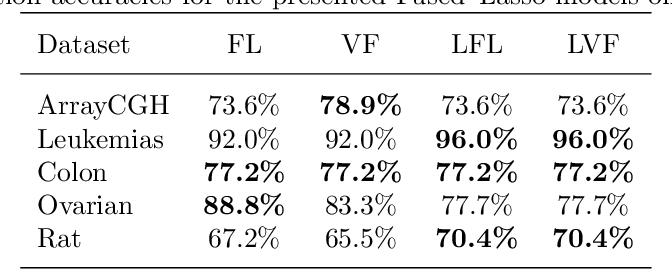We study \emph{TV regularization}, a widely used technique for eliciting structured sparsity. In particular, we propose efficient algorithms for computing prox-operators for $\ell_p$-norm TV. The most important among these is $\ell_1$-norm TV, for whose prox-operator we present a new geometric analysis which unveils a hitherto unknown connection to taut-string methods. This connection turns out to be remarkably useful as it shows how our geometry guided implementation results in efficient weighted and unweighted 1D-TV solvers, surpassing state-of-the-art methods. Our 1D-TV solvers provide the backbone for building more complex (two or higher-dimensional) TV solvers within a modular proximal optimization approach. We review the literature for an array of methods exploiting this strategy, and illustrate the benefits of our modular design through extensive suite of experiments on (i) image denoising, (ii) image deconvolution, (iii) four variants of fused-lasso, and (iv) video denoising. To underscore our claims and permit easy reproducibility, we provide all the reviewed and our new TV solvers in an easy to use multi-threaded C++, Matlab and Python library.

* 67 pages, 32 figures, new non-iterative fast TV algorithm, extensive new experiments, corresponds to the github proxtv repository now
Click to Read Paper and Get Code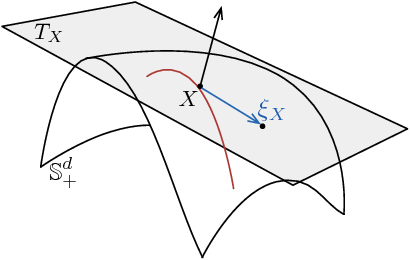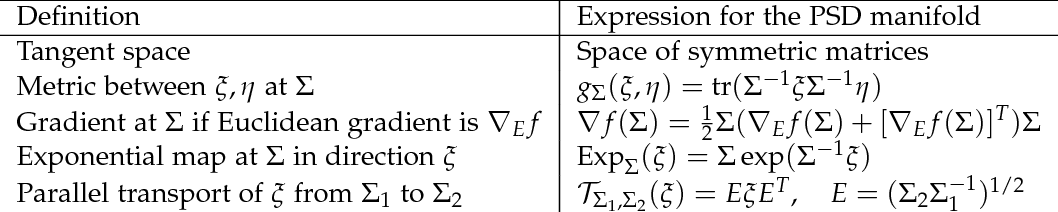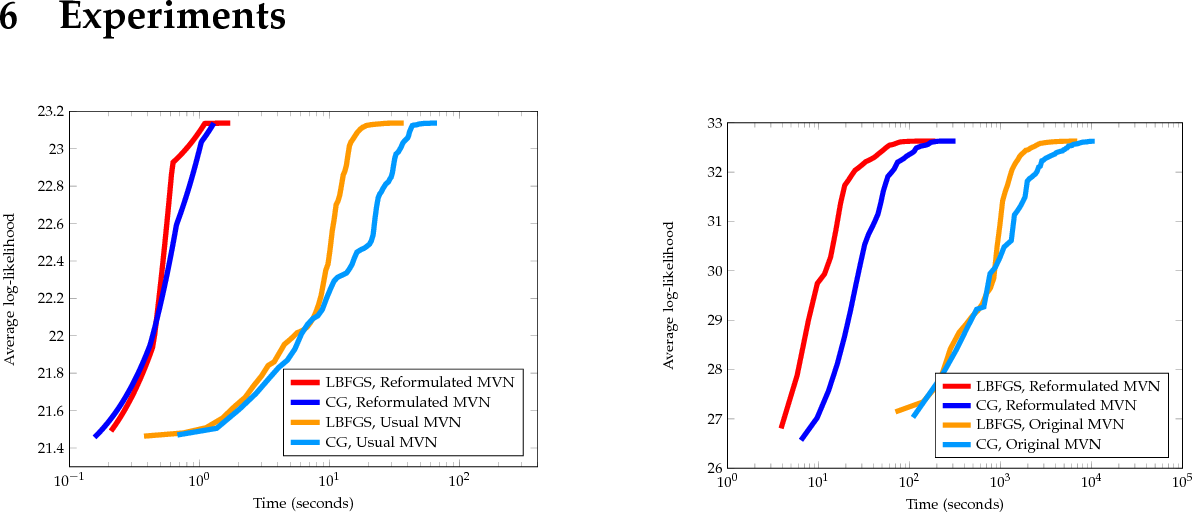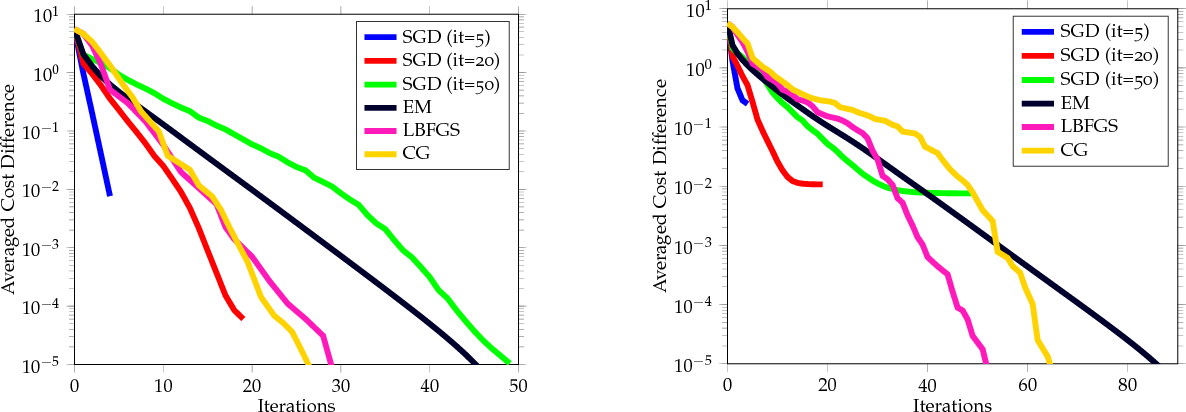We consider maximum likelihood estimation for Gaussian Mixture Models (Gmms). This task is almost invariably solved (in theory and practice) via the Expectation Maximization (EM) algorithm. EM owes its success to various factors, of which is its ability to fulfill positive definiteness constraints in closed form is of key importance. We propose an alternative to EM by appealing to the rich Riemannian geometry of positive definite matrices, using which we cast Gmm parameter estimation as a Riemannian optimization problem. Surprisingly, such an out-of-the-box Riemannian formulation completely fails and proves much inferior to EM. This motivates us to take a closer look at the problem geometry, and derive a better formulation that is much more amenable to Riemannian optimization. We then develop (Riemannian) batch and stochastic gradient algorithms that outperform EM, often substantially. We provide a non-asymptotic convergence analysis for our stochastic method, which is also the first (to our knowledge) such global analysis for Riemannian stochastic gradient. Numerous empirical results are included to demonstrate the effectiveness of our methods.

* 21 pages, 6 figures
Click to Read Paper and Get Code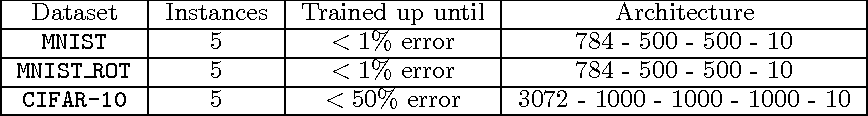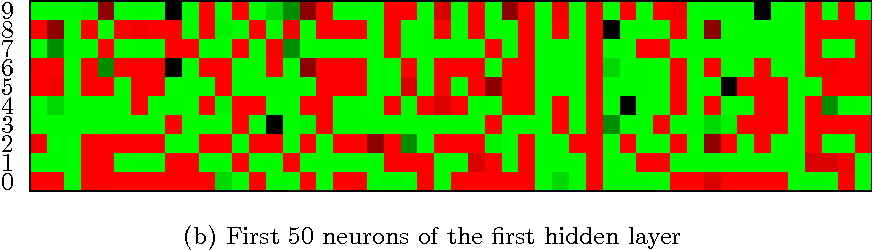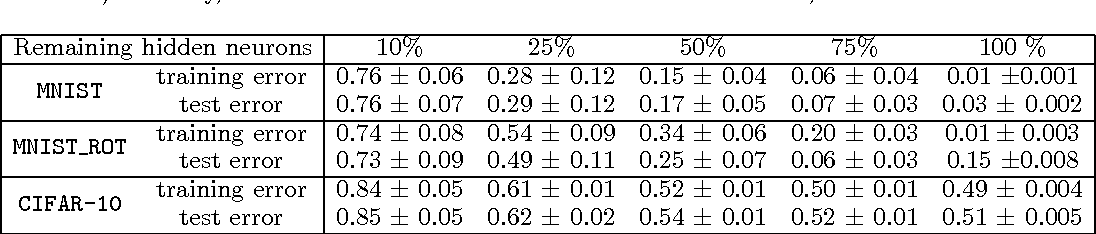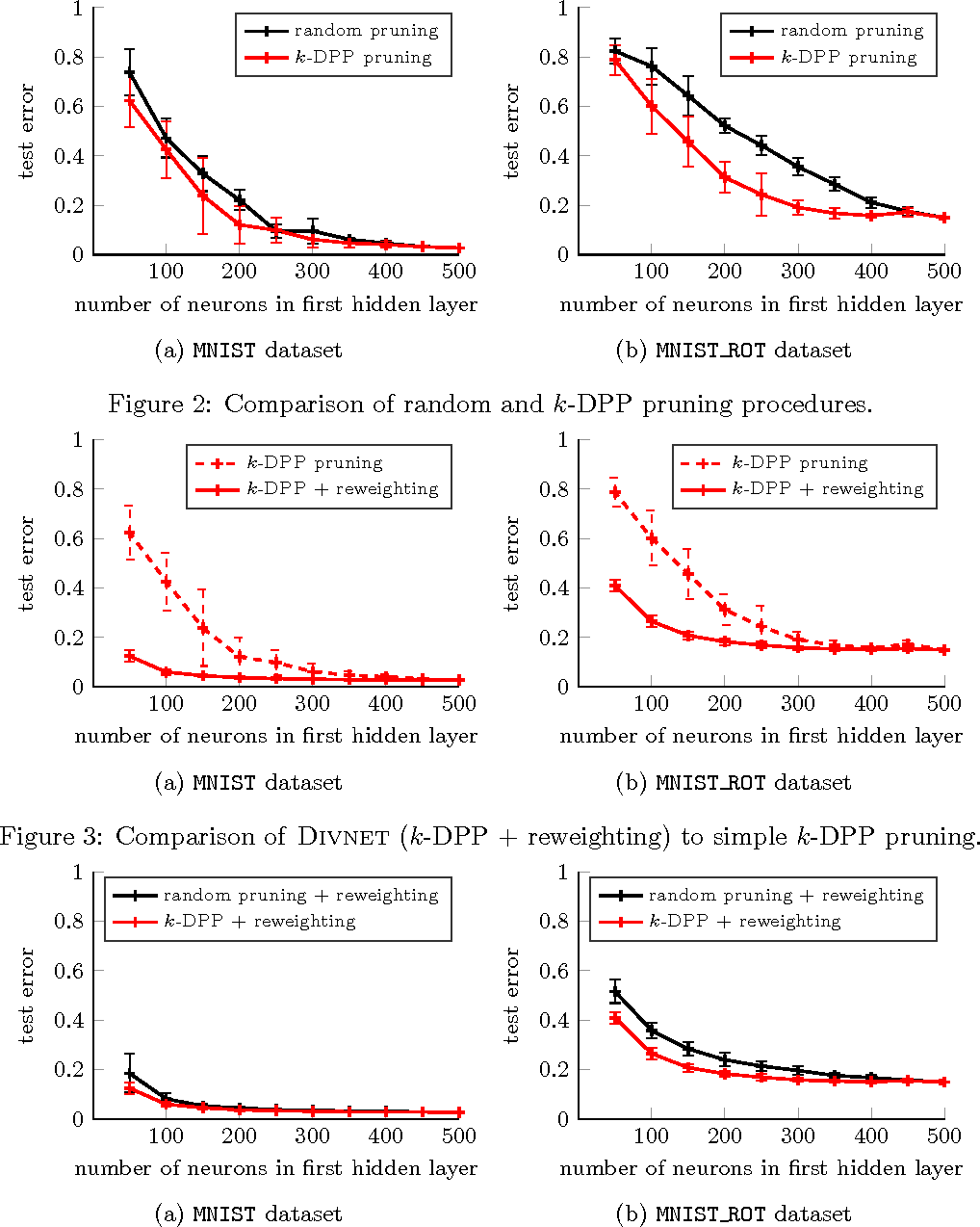We introduce Divnet, a flexible technique for learning networks with diverse neurons. Divnet models neuronal diversity by placing a Determinantal Point Process (DPP) over neurons in a given layer. It uses this DPP to select a subset of diverse neurons and subsequently fuses the redundant neurons into the selected ones. Compared with previous approaches, Divnet offers a more principled, flexible technique for capturing neuronal diversity and thus implicitly enforcing regularization. This enables effective auto-tuning of network architecture and leads to smaller network sizes without hurting performance. Moreover, through its focus on diversity and neuron fusing, Divnet remains compatible with other procedures that seek to reduce memory footprints of networks. We present experimental results to corroborate our claims: for pruning neural networks, Divnet is seen to be notably superior to competing approaches.

* This paper appeared under the shorter title Diversity Networks at ICLR 2016 (http://www.iclr.cc/doku.php?id=iclr2016:main#accepted_papers_conference_track)
Click to Read Paper and Get Code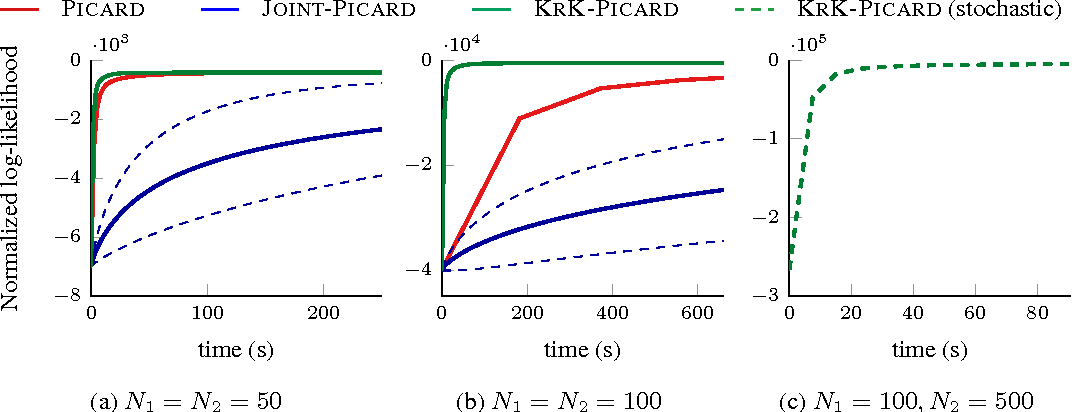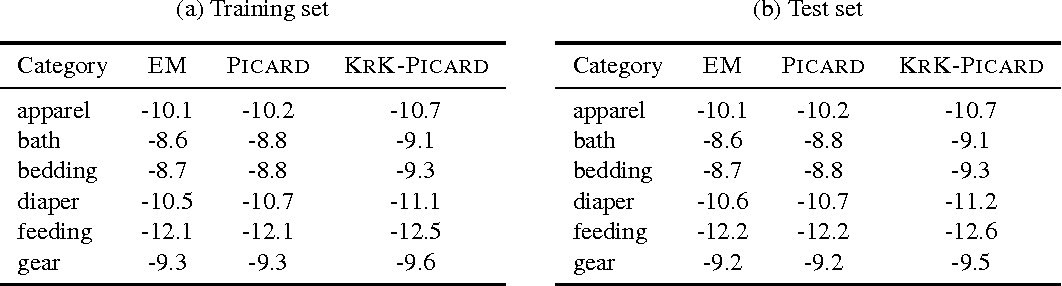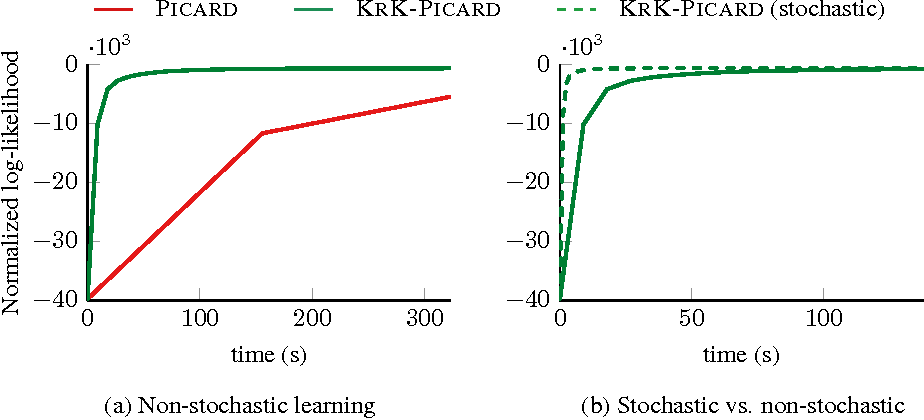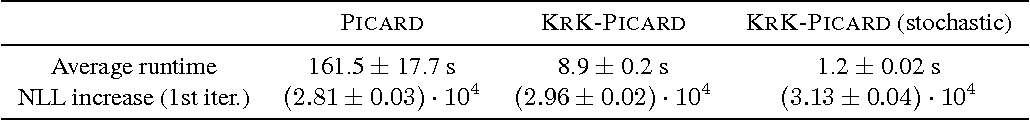Determinantal Point Processes (DPPs) are probabilistic models over all subsets a ground set of $N$ items. They have recently gained prominence in several applications that rely on "diverse" subsets. However, their applicability to large problems is still limited due to the $\mathcal O(N^3)$ complexity of core tasks such as sampling and learning. We enable efficient sampling and learning for DPPs by introducing KronDPP, a DPP model whose kernel matrix decomposes as a tensor product of multiple smaller kernel matrices. This decomposition immediately enables fast exact sampling. But contrary to what one may expect, leveraging the Kronecker product structure for speeding up DPP learning turns out to be more difficult. We overcome this challenge, and derive batch and stochastic optimization algorithms for efficiently learning the parameters of a KronDPP.

Click to Read Paper and Get Code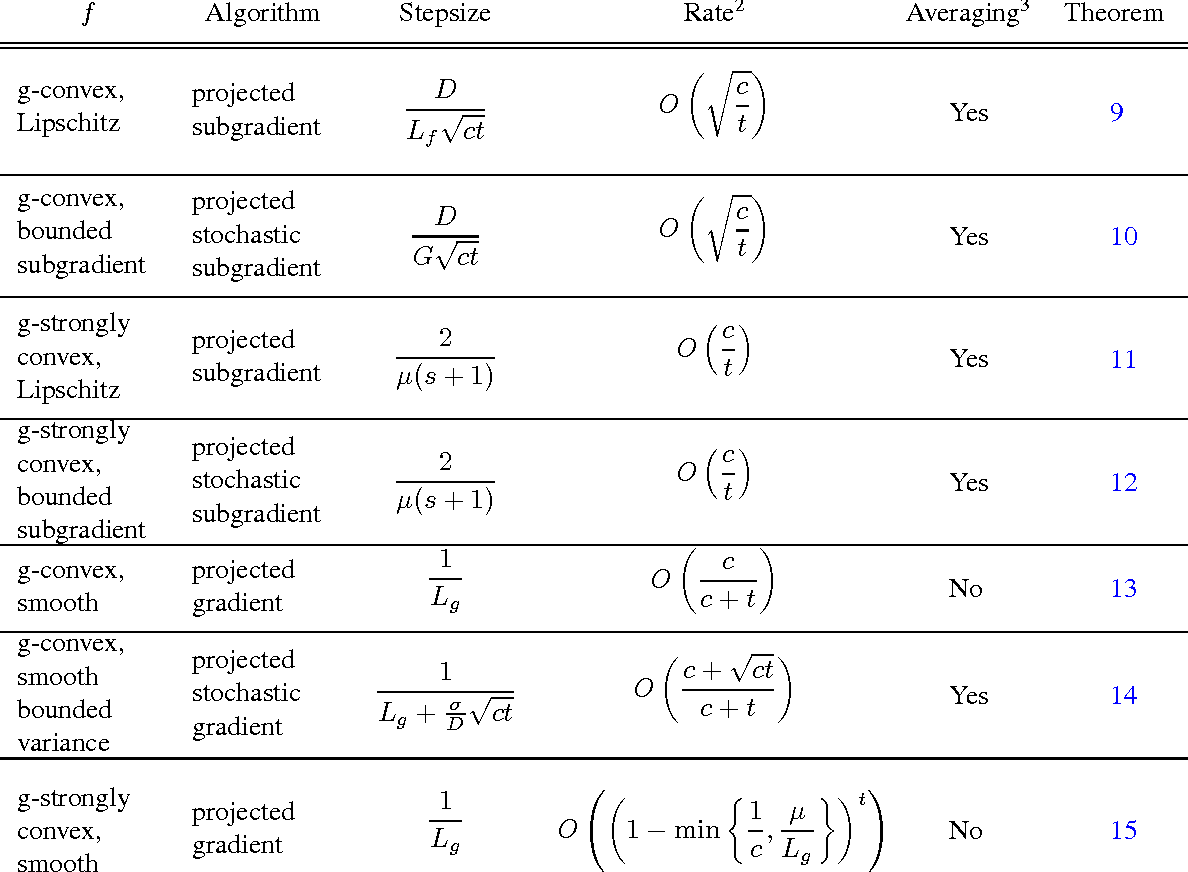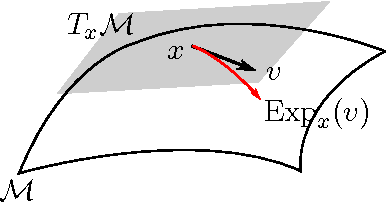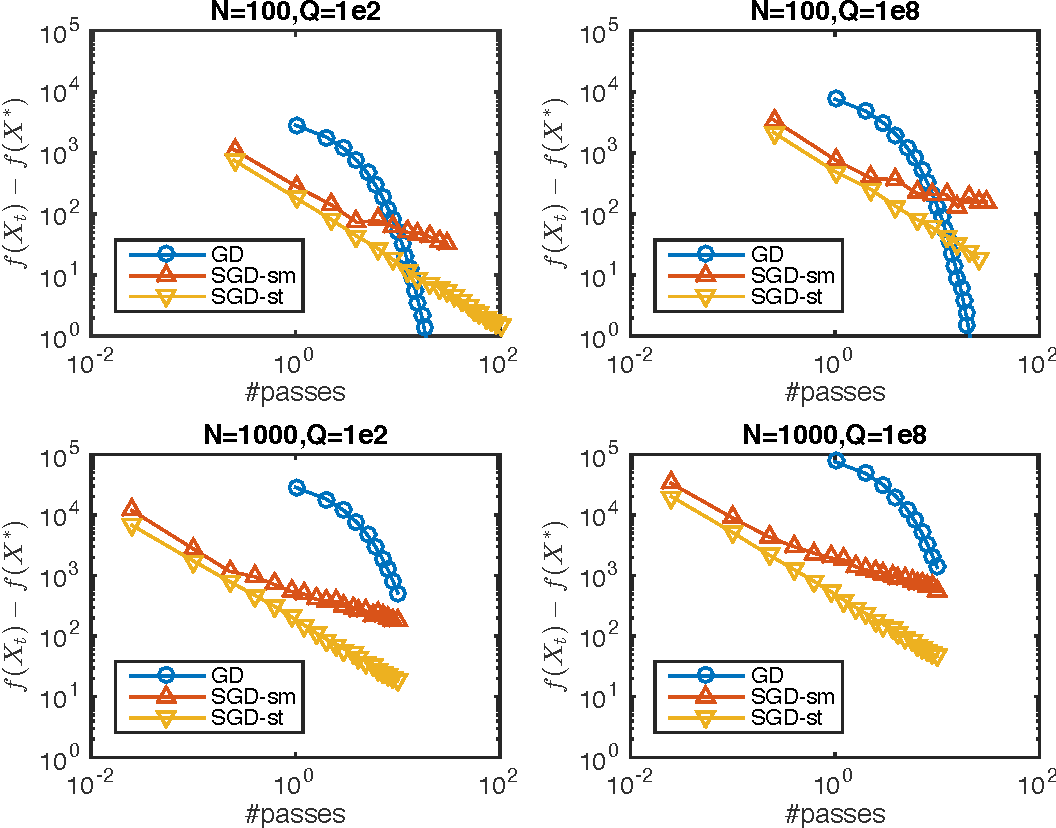Geodesic convexity generalizes the notion of (vector space) convexity to nonlinear metric spaces. But unlike convex optimization, geodesically convex (g-convex) optimization is much less developed. In this paper we contribute to the understanding of g-convex optimization by developing iteration complexity analysis for several first-order algorithms on Hadamard manifolds. Specifically, we prove upper bounds for the global complexity of deterministic and stochastic (sub)gradient methods for optimizing smooth and nonsmooth g-convex functions, both with and without strong g-convexity. Our analysis also reveals how the manifold geometry, especially \emph{sectional curvature}, impacts convergence rates. To the best of our knowledge, our work is the first to provide global complexity analysis for first-order algorithms for general g-convex optimization.

* 21 pages
Click to Read Paper and Get Code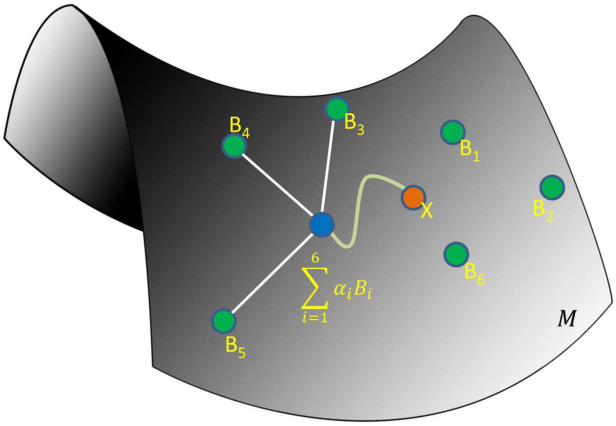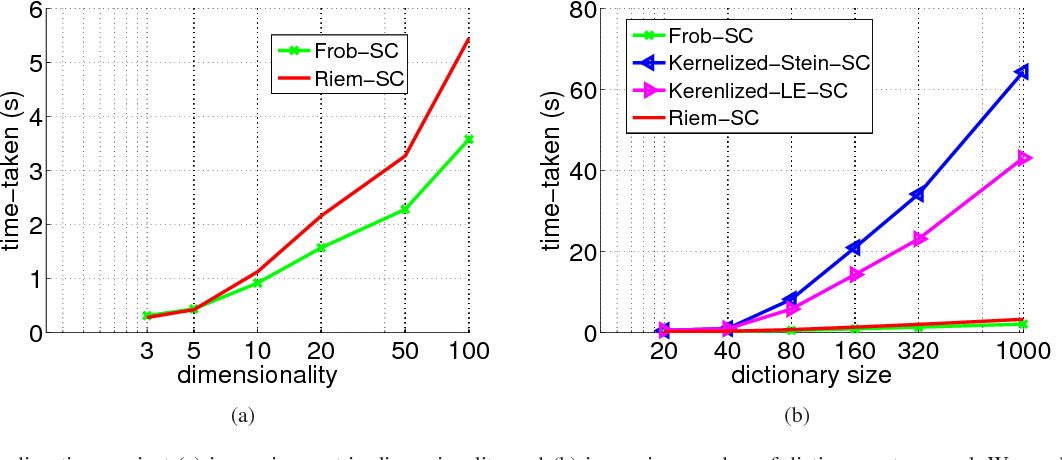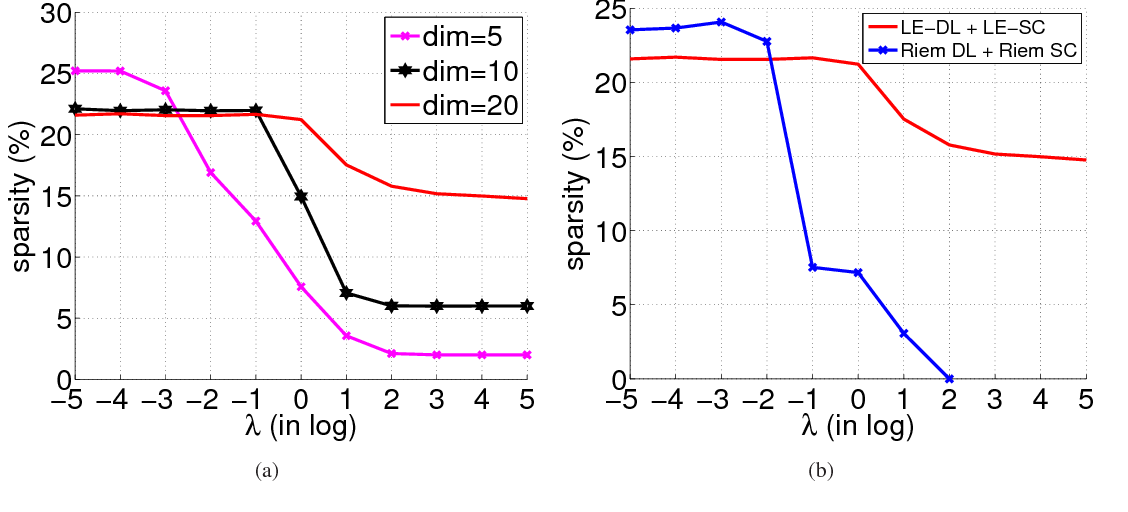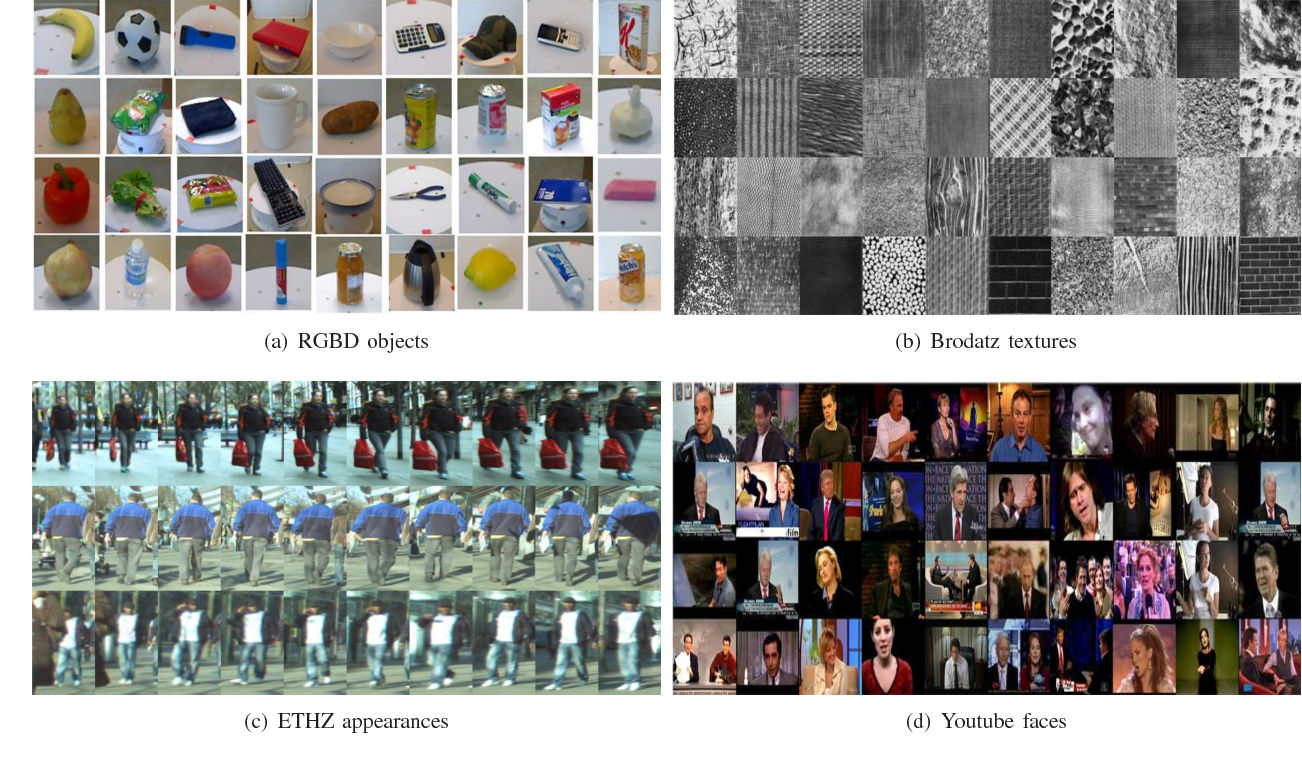Data encoded as symmetric positive definite (SPD) matrices frequently arise in many areas of computer vision and machine learning. While these matrices form an open subset of the Euclidean space of symmetric matrices, viewing them through the lens of non-Euclidean Riemannian geometry often turns out to be better suited in capturing several desirable data properties. However, formulating classical machine learning algorithms within such a geometry is often non-trivial and computationally expensive. Inspired by the great success of dictionary learning and sparse coding for vector-valued data, our goal in this paper is to represent data in the form of SPD matrices as sparse conic combinations of SPD atoms from a learned dictionary via a Riemannian geometric approach. To that end, we formulate a novel Riemannian optimization objective for dictionary learning and sparse coding in which the representation loss is characterized via the affine invariant Riemannian metric. We also present a computationally simple algorithm for optimizing our model. Experiments on several computer vision datasets demonstrate superior classification and retrieval performance using our approach when compared to sparse coding via alternative non-Riemannian formulations.

Click to Read Paper and Get Code
Determinantal point processes (DPPs) offer an elegant tool for encoding probabilities over subsets of a ground set. Discrete DPPs are parametrized by a positive semidefinite matrix (called the DPP kernel), and estimating this kernel is key to learning DPPs from observed data. We consider the task of learning the DPP kernel, and develop for it a surprisingly simple yet effective new algorithm. Our algorithm offers the following benefits over previous approaches: (a) it is much simpler; (b) it yields equally good and sometimes even better local maxima; and (c) it runs an order of magnitude faster on large problems. We present experimental results on both real and simulated data to illustrate the numerical performance of our technique.

* ICML, 2015
Click to Read Paper and Get Code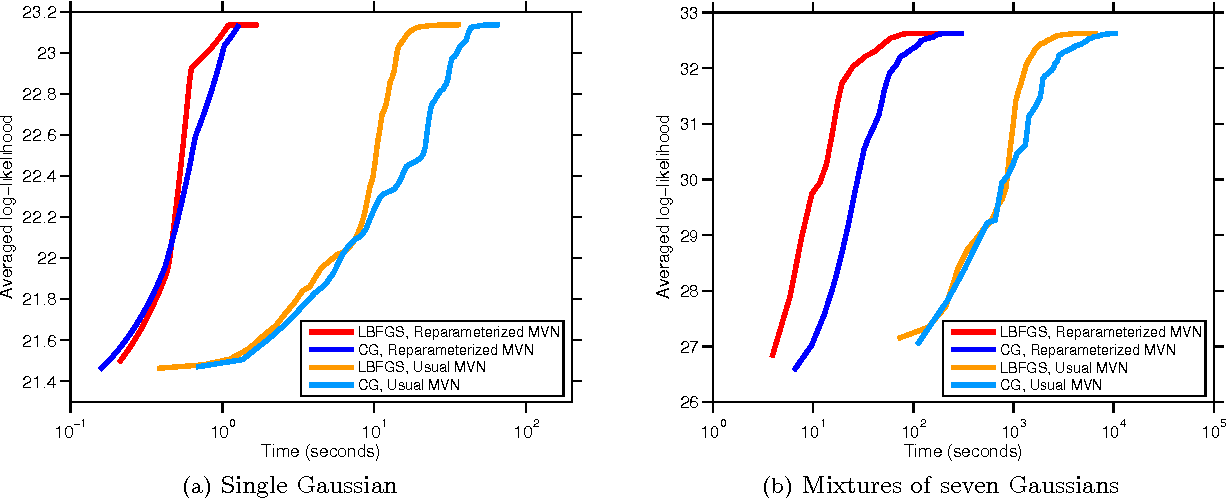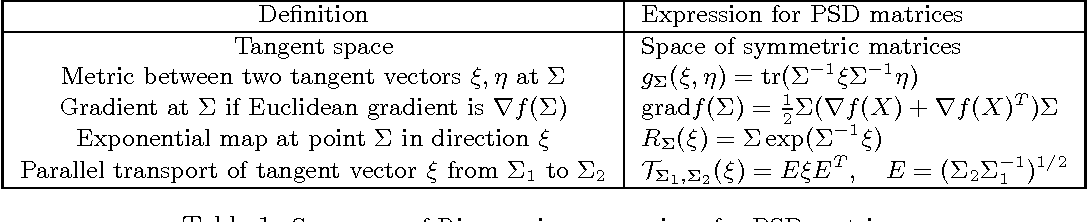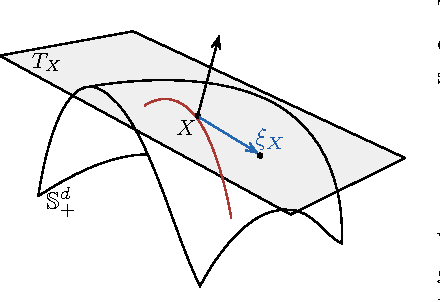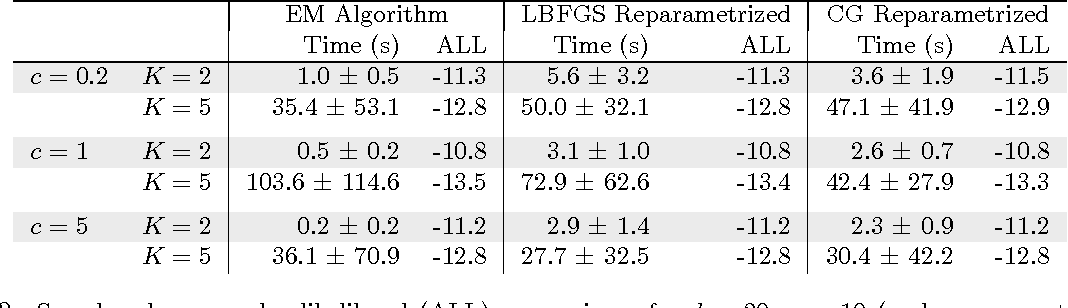We take a new look at parameter estimation for Gaussian Mixture Models (GMMs). In particular, we propose using \emph{Riemannian manifold optimization} as a powerful counterpart to Expectation Maximization (EM). An out-of-the-box invocation of manifold optimization, however, fails spectacularly: it converges to the same solution but vastly slower. Driven by intuition from manifold convexity, we then propose a reparamerization that has remarkable empirical consequences. It makes manifold optimization not only match EM---a highly encouraging result in itself given the poor record nonlinear programming methods have had against EM so far---but also outperform EM in many practical settings, while displaying much less variability in running times. We further highlight the strengths of manifold optimization by developing a somewhat tuned manifold LBFGS method that proves even more competitive and reliable than existing manifold optimization tools. We hope that our results encourage a wider consideration of manifold optimization for parameter estimation problems.

* 19 pages
Click to Read Paper and Get Code
Large graphs abound in machine learning, data mining, and several related areas. A useful step towards analyzing such graphs is that of obtaining certain summary statistics - e.g., or the expected length of a shortest path between two nodes, or the expected weight of a minimum spanning tree of the graph, etc. These statistics provide insight into the structure of a graph, and they can help predict global properties of a graph. Motivated thus, we propose to study statistical properties of structured subgraphs (of a given graph), in particular, to estimate the expected objective function value of a combinatorial optimization problem over these subgraphs. The general task is very difficult, if not unsolvable; so for concreteness we describe a more specific statistical estimation problem based on spanning trees. We hope that our position paper encourages others to also study other types of graphical structures for which one can prove nontrivial statistical estimates.

* Paper for the NIPS Workshop on Discrete Optimization for Machine Learning (DISCML) (2011): Uncertainty, Generalization and Feedback
Click to Read Paper and Get Code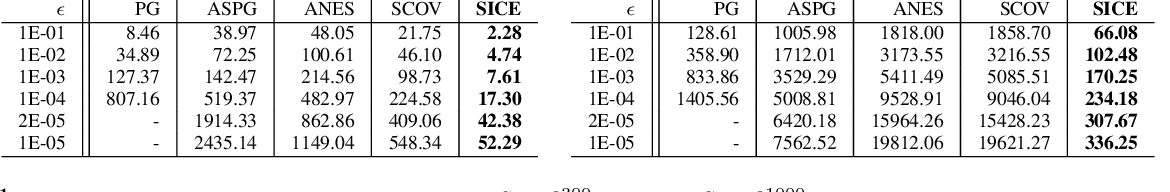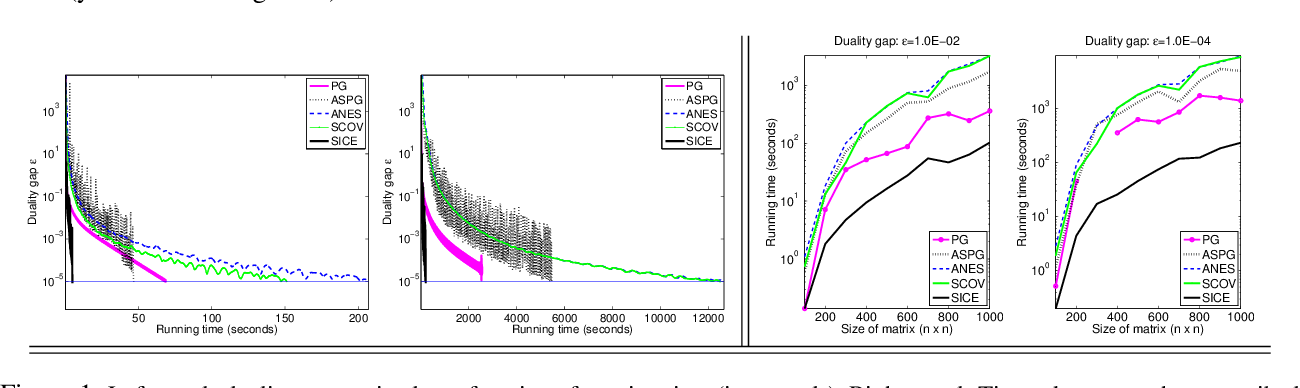We study the problem of estimating from data, a sparse approximation to the inverse covariance matrix. Estimating a sparsity constrained inverse covariance matrix is a key component in Gaussian graphical model learning, but one that is numerically very challenging. We address this challenge by developing a new adaptive gradient-based method that carefully combines gradient information with an adaptive step-scaling strategy, which results in a scalable, highly competitive method. Our algorithm, like its predecessors, maximizes an $\ell_1$-norm penalized log-likelihood and has the same per iteration arithmetic complexity as the best methods in its class. Our experiments reveal that our approach outperforms state-of-the-art competitors, often significantly so, for large problems.

* 13 pages
Click to Read Paper and Get Code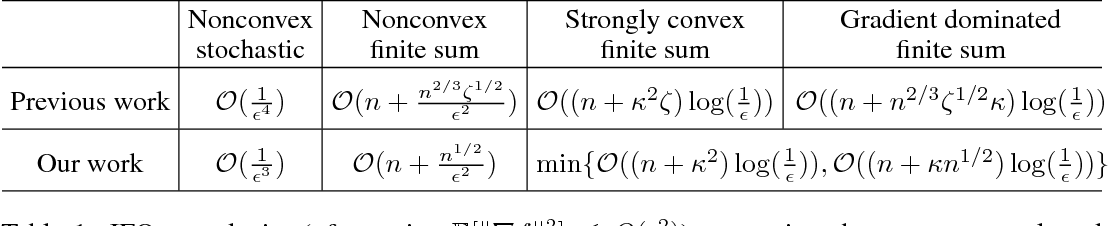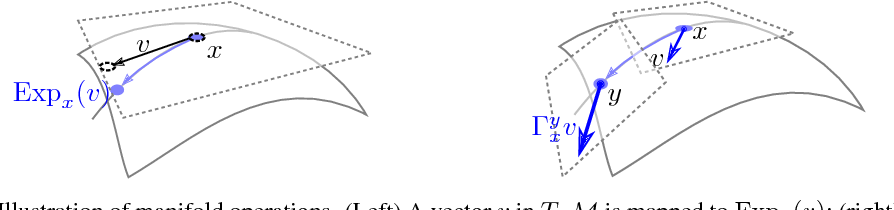We study smooth stochastic optimization problems on Riemannian manifolds. Via adapting the recently proposed SPIDER algorithm \citep{fang2018spider} (a variance reduced stochastic method) to Riemannian manifold, we can achieve faster rate than known algorithms in both the finite sum and stochastic settings. Unlike previous works, by \emph{not} resorting to bounding iterate distances, our analysis yields curvature independent convergence rates for both the nonconvex and strongly convex cases.

* arXiv admin note: text overlap with arXiv:1605.07147
Click to Read Paper and Get Code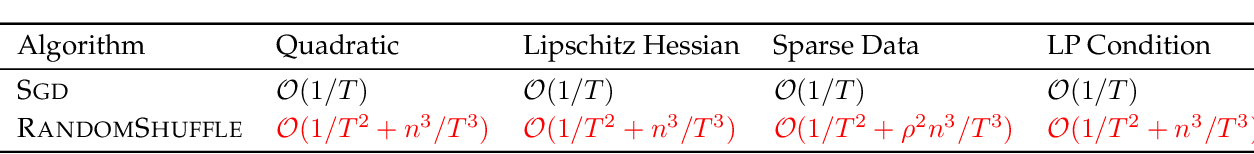A long-standing problem in the theory of stochastic gradient descent (SGD) is to prove that its without-replacement version RandomShuffle converges faster than the usual with-replacement version. We present the first (to our knowledge) non-asymptotic solution to this problem, which shows that after a "reasonable" number of epochs RandomShuffle indeed converges faster than SGD. Specifically, we prove that under strong convexity and second-order smoothness, the sequence generated by RandomShuffle converges to the optimal solution at the rate O(1/T^2 + n^3/T^3), where n is the number of components in the objective, and T is the total number of iterations. This result shows that after a reasonable number of epochs RandomShuffle is strictly better than SGD (which converges as O(1/T)). The key step toward showing this better dependence on T is the introduction of n into the bound; and as our analysis will show, in general a dependence on n is unavoidable without further changes to the algorithm. We show that for sparse data RandomShuffle has the rate O(1/T^2), again strictly better than SGD. Furthermore, we discuss extensions to nonconvex gradient dominated functions, as well as non-strongly convex settings.

Click to Read Paper and Get Code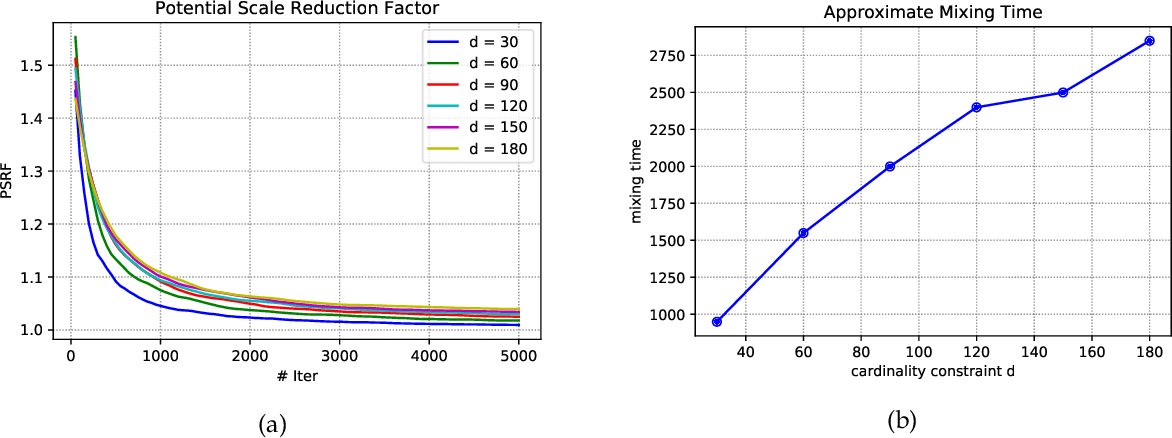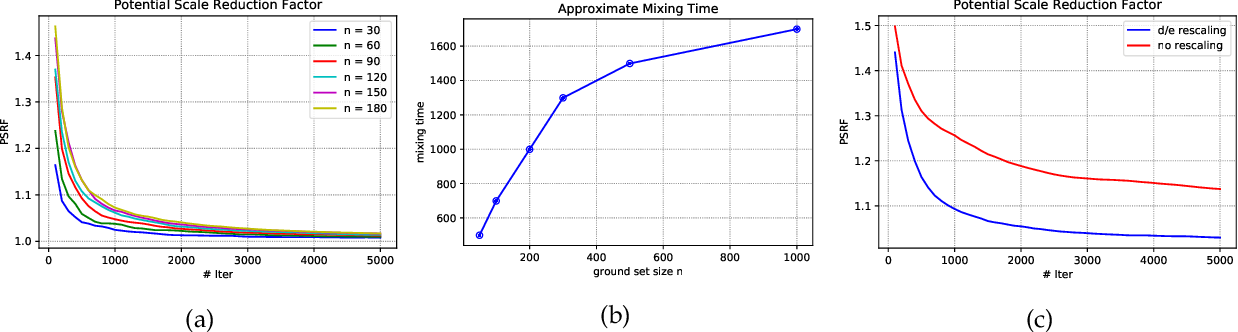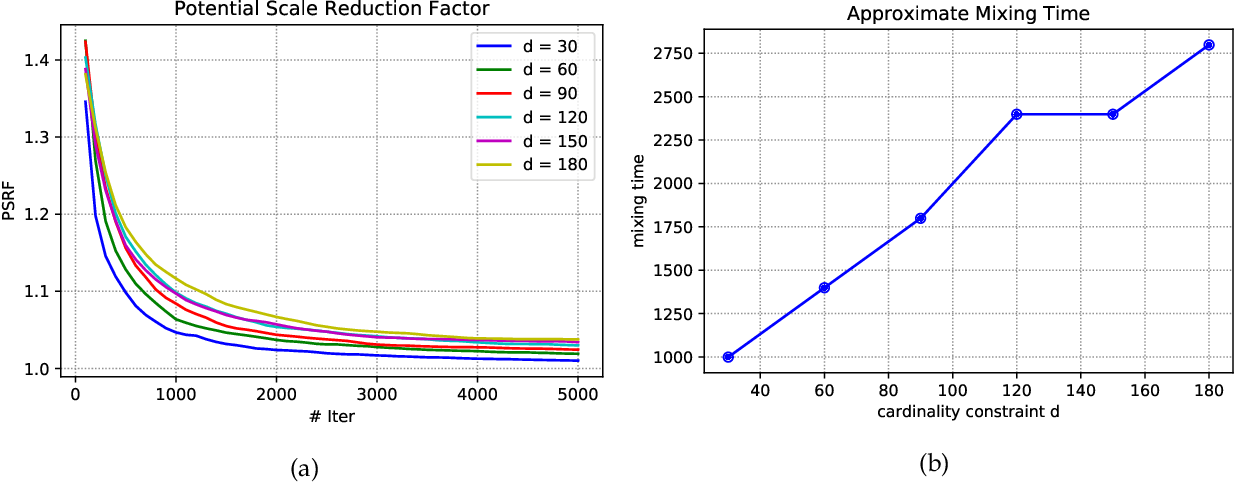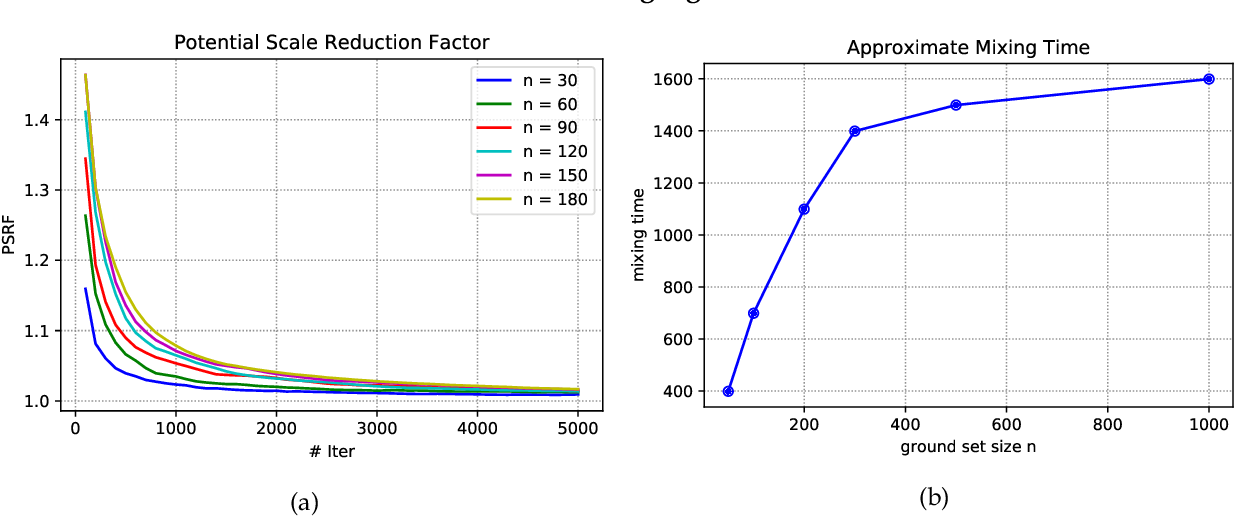Strongly log-concave (SLC) distributions are a rich class of discrete probability distributions over subsets of some ground set. They are strictly more general than strongly Rayleigh (SR) distributions such as the well-known determinantal point process. While SR distributions offer elegant models of diversity, they lack an easy control over how they express diversity. We propose SLC as the right extension of SR that enables easier, more intuitive control over diversity, illustrating this via examples of practical importance. We develop two fundamental tools needed to apply SLC distributions to learning and inference: sampling and mode finding. For sampling we develop an MCMC sampler and give theoretical mixing time bounds. For mode finding, we establish a weak log-submodularity property for SLC functions and derive optimization guarantees for a distorted greedy algorithm.

Click to Read Paper and Get Code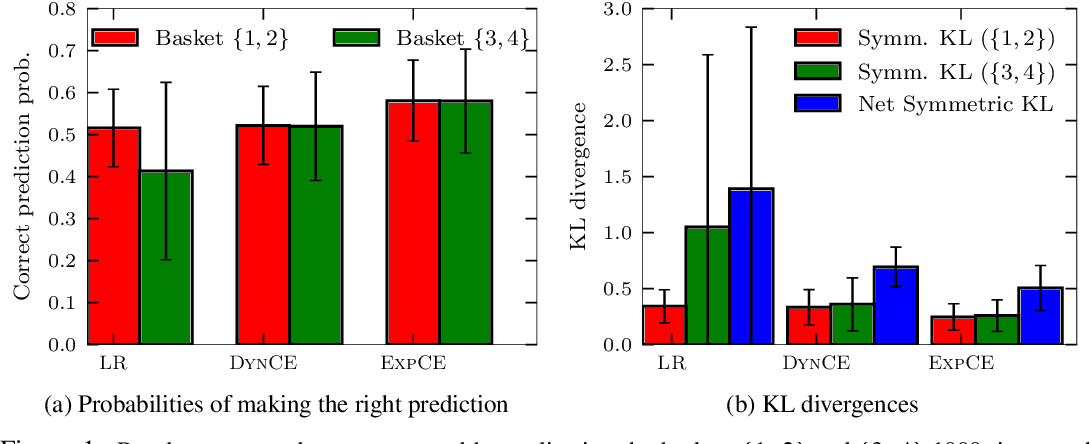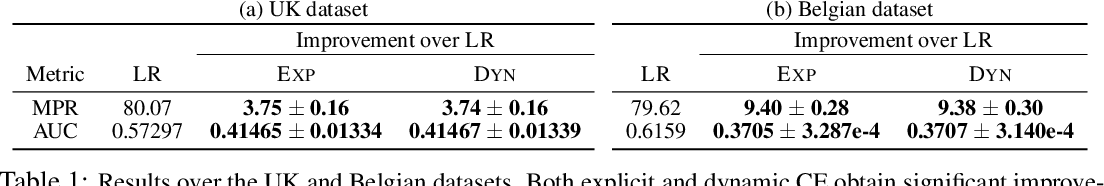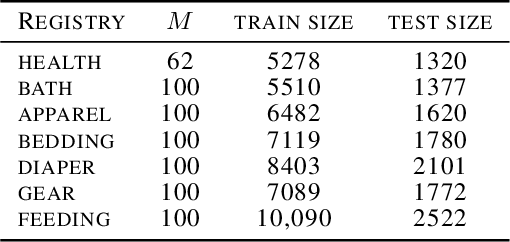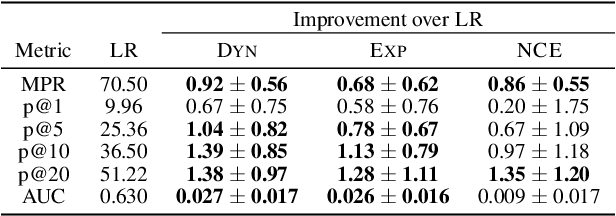Determinantal Point Processes (DPPs) have attracted significant interest from the machine-learning community due to their ability to elegantly and tractably model the delicate balance between quality and diversity of sets. We consider learning DPPs from data, a key task for DPPs; for this task, we introduce a novel optimization problem, Contrastive Estimation (CE), which encodes information about "negative" samples into the basic learning model. CE is grounded in the successful use of negative information in machine-vision and language modeling. Depending on the chosen negative distribution (which may be static or evolve during optimization), CE assumes two different forms, which we analyze theoretically and experimentally. We evaluate our new model on real-world datasets; on a challenging dataset, CE learning delivers a considerable improvement in predictive performance over a DPP learned without using contrastive information.

Click to Read Paper and Get Code